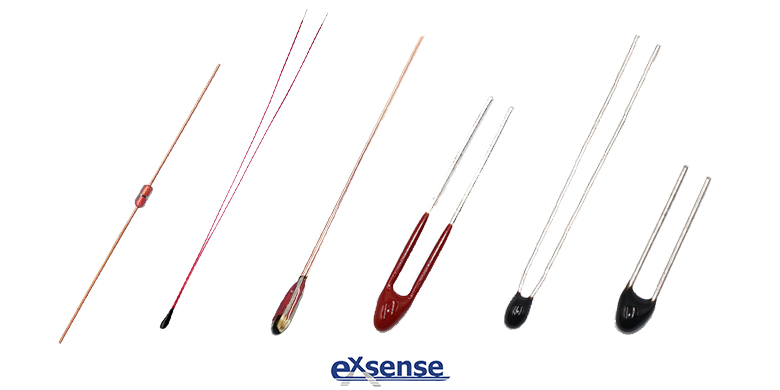QQCustomer Service
Product Catalogue
Tel :
+86-758-2661333
+86-15218448949
Fax :
+86-758-2698912
QQ :
1919442378
No. 201, Shunjing Road, The First Community, Guicheng Street, Dinghu District, Zhaoqing, China, 526070
Technical Support
Technical Support
Linear Compensation Circuit of NTC Thermistor Based On Output Characteristics of Bipolar Junction Transistor-3
2019/01/24 03:01:47The method of thermistor linear compensation circuit based on transistor output characteristic is to effectively overcome the nonlinear relationship between the resistance value and temperature of the thermistor by applying a suitable linear compensation circuit to the NTC thermistor, and to realize the linear change relationship between the output result and the temperature in the process of thermistor temperature measurement.

The method uses the nonlinearity of the first NPN type transistor Q1 output characteristic curve to counteract the nonlinearity between the resistance value and temperature of NTC thermistor R mutually, that is, the nonlinearity of the first NPN type transistor Q1 is used to compensate the nonlinearity of NTC Thermistor R, and finally the linear relationship between the output voltage V and the temperature T is reached.

There is a serious nonlinear relationship between the resistance value and temperature of NTC thermistor R. With the increase of temperature, the resistance value of NTC thermistor R will decrease, and the practical research shows that the nonlinear relationship between the resistance value and temperature of NTC Thermistor R is the e exponential relationship. Common BJT transistor, there are three working states with amplification, cutoff and saturation. By adjusting the size relationship among the collector current IC, the collector and the emitter voltage V and the base current I, the working state of the BJT transistor can be controlled. In the case of the base current I fixed, similar to the thermistor, the BJT transistor collector current I and the voltage V between the collector and the emitter also have a very serious nonlinearity, and this nonlinear relationship satisfies the e exponent, this nonlinear relationship will also be controlled by the base current I.

In addition, the output voltage V of thermistor linear compensation circuit based on transistor output characteristic is the collector emitter voltage V of the first NPN type transistor Q1.

The thermistor linear compensation circuit based on transistor output characteristic of this method, when the circuit is working normally, the change of sensing temperature T will change the resistance value R of the thermistor, so the output voltage V will also change. However, in any case, the circuit can always ensure a great linear relationship between the output voltage V and the temperature T sensing by the NTC thermistor, and the linear compensation effect is shown in the triangular curve in the figure. In order to further verify the validity of the circuit from the reverse, using the third constant resistor R3 instead of the first NPN transistor Q1 in the Compensation Module 4, the circuit will lose its linearization effect, as shown in the dot curve in the figure.Based on the above theory, the thermistor linear compensation circuit based on transistor output characteristic proposed by this method is shown in the figure, its fundamental principle is to use the e exponential nonlinear relationship of BJT output characteristic curve to counteract the nonlinear relationship of e exponential of NTC thermistor R to achieve the goal of linear compensation mutually.

The thermistor linear compensation circuit based on transistor output characteristic proposed by this method, which effectively overcomes the nonlinear relationship between the resistance value and temperature of the thermistor by applying a suitable linear compensation circuit to the NTC thermistor, and realizes the linear change relationship between the output result and the temperature during the temperature measurement of the NTC thermistor. In practical applications, the first constant resistor R1, the second constant resistor R2 and the load resistor R can be replaced with adjustable resistors, thus facilitating the debugging and optimization of the circuit easily.

mqu.cn site.nuo.cn